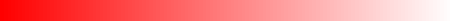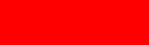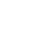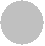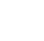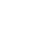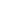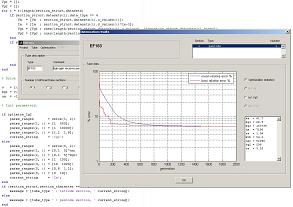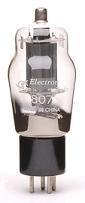Jeroen's
Jeroen's
Technical Corner
Technical Corner
Simulation software, tube electronics and more
Main

Motega
Mathematics of tube models
 The mathematical models used in Motega are based on work performed by Norman Koren. I only made a minor modification to his tube models, which gave me a slightly better result. It should be noted that I do not pursue a 'perfect' tube model. For allmost every tube that you may use, the tube models generated by Motega are accurate within a few percent and that's more than enough for my (and likely your) purposes. Keep in mind that datasheets only describe a typical behavior of the tube in question: if you take 10 new tubes then you'll find that none of them will fit the datasheet within a few percent... In the following 3 sections I'll give the mathematical models for diodes, triodes and tetrodes/pentodes. Because there is already so much said about this topic on the internet, I will not elaborate on these models further here. Diode model Motega uses a diode model which consists of an ideal 'lossless' diode in series with a resistor r. The voltage across the Motega diode model therefore consists of two components: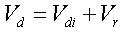The anode current of the ideal diode is given by: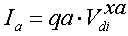If the voltage drop across the resistor in the Motega diode model is substituted, then the following equation for the anode current is obtained: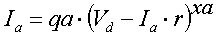There is no analytical solution for this equation, so the anode current has to be calculated in an iterative process. Triode model Calculation of the triode anode current starts with making the exponential factor xa dependent of the anode voltage Va. This is in fact the only modification I made to the original model of Norman Koren: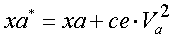Two intermediate calculations are made first, given by: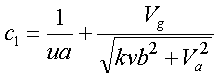and by: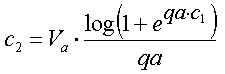Finally, the anode current is given by: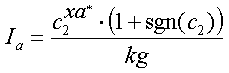Tetrode/pentode model Equivalent to the calculations of the triode anode current, calculation of the tetrode/pentode anode current starts with making the exponential factor xa dependent of the screen grid voltage Vg2: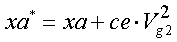Again two intermediate calculations are made first, given by: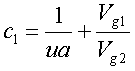and by: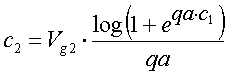Finally, the anode current is given by: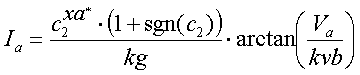The screen grid current Ig2 is calculated by using almost the same model as the anode current, only the exponential factor xa does not depent on the screen grid voltage. This can be obtained by setting ce=0 in the anode current model.